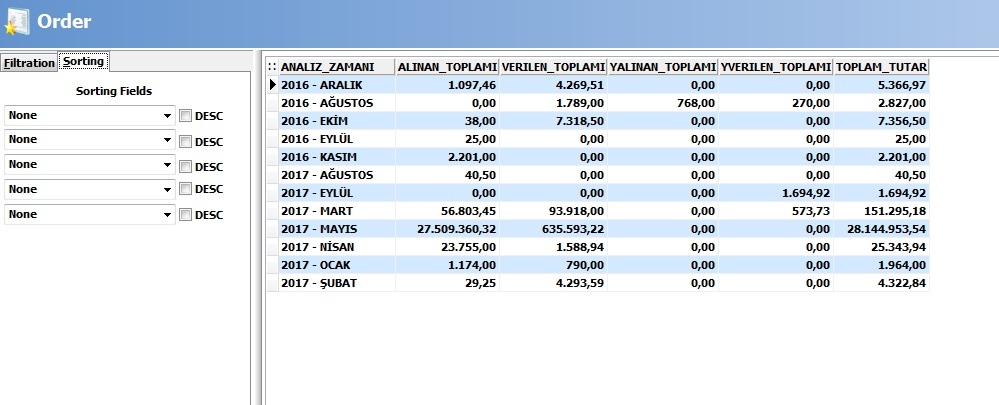CODE: A2842

### Wolvox ERP Month Based Order Analysis

In the Wolvox ERP program you can get a report on screenshot down below by using "Custom Report Transaction" section, With this report, you can perform a general analysis of your orders on the monthly basis.To get a report, first enter the "Custom Report Transaction" and press "Add New Report" button. Type the name for report, copy SQL command in down bellow to the SQL code field and press save button.

SQL KODU :

SELECT * FROM     (                 SELECT   (EXTRACT(YEAR FROM TARIHI) || ' - ' || CASE WHEN EXTRACT(MONTH FROM TARIHI)=1 THEN 'OCAK'  ELSE
CASE WHEN EXTRACT(MONTH FROM TARIHI)=2 THEN 'ŞUBAT' ELSE
CASE WHEN EXTRACT(MONTH FROM TARIHI)=3 THEN 'MART' ELSE
CASE WHEN EXTRACT(MONTH FROM TARIHI)=4 THEN 'NİSAN' ELSE
CASE WHEN EXTRACT(MONTH FROM TARIHI)=5 THEN 'MAYIS' ELSE
CASE WHEN EXTRACT(MONTH FROM TARIHI)=6 THEN 'HAZİRAN' ELSE
CASE WHEN EXTRACT(MONTH FROM TARIHI)=7 THEN 'TEMMUZ' ELSE
CASE WHEN EXTRACT(MONTH FROM TARIHI)=8 THEN 'AĞUSTOS' ELSE
CASE WHEN EXTRACT(MONTH FROM TARIHI)=9 THEN 'EYLÜL' ELSE
CASE WHEN EXTRACT(MONTH FROM TARIHI)=10 THEN 'EKİM' ELSE
CASE WHEN EXTRACT(MONTH FROM TARIHI)=11 THEN 'KASIM' ELSE
CASE WHEN EXTRACT(MONTH FROM TARIHI)=12 THEN 'ARALIK' END  END END END END END END END END END END END) ANALIZ_ZAMANI,

SUM(CASE WHEN SIPARIS_TURU=1 THEN TOPLAM_ARA_KPB ELSE 0 END) as ALINAN_TOPLAMI,
SUM(CASE WHEN SIPARIS_TURU=2 THEN TOPLAM_ARA_KPB ELSE 0 END) as VERILEN_TOPLAMI,
SUM(CASE WHEN SIPARIS_TURU=3 THEN TOPLAM_ARA_KPB ELSE 0 END) as YALINAN_TOPLAMI,
SUM(CASE WHEN SIPARIS_TURU=4 THEN TOPLAM_ARA_KPB ELSE 0 END) as YVERILEN_TOPLAMI,
SUM(TOPLAM_ARA_KPB) TOPLAM_TUTAR FROM SIPARIS
WHERE TOPLAM_ARA_KPB > 0
AND SILINDI='0'

GROUP BY 1  ) SONUC

ORDER BY ANALIZ_ZAMANI